## Introduction

The authors of the paper propose a novel way to counter catastrophic forgetting during sequential learning of GANs. They propose Memory Replay GANs (MeRGANs), a conditional GAN that integrates memory replay.

## Sequential learning

The task proposed by the authors is to sequentially learn from a training set $$S = \{ S_1, ..., S_M\}$$ where M is the number of categories. Each subset $$S_C$$ represents the training set for a specific category and is considered a task t. The goal is to train a conditional GAN to generate images from all the sets after being trained on each set sequentially.

All methods use an AC-GAN framework with the WGAN-GP loss.

The generator’s parameters are denoted $$\theta^G$$. The generator generates images according to $$\tilde{x} = G_{\theta^G}(z, c)$$ where z is a latent vector and c is a category.

The discriminator’s parameters are denoted $$\theta^D$$.

The AC-GAN uses a auxiliary classifier C whose parameters are denotes $$\theta^C$$. The classifier predicts the image labels $$\tilde{c} = C_{\theta^C}(x)$$

The GAN’s parameters are denoted as $$\theta = (\theta^G, \theta^D, \theta^C)$$ in the equations.

## Previous Methods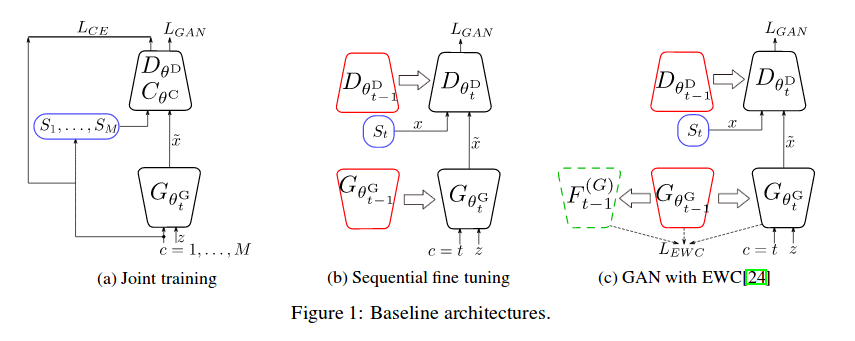Joint learning

This method is not sequential learning, all categories are learned at the same time. The classical GAN optimization is used with WGAN modifications.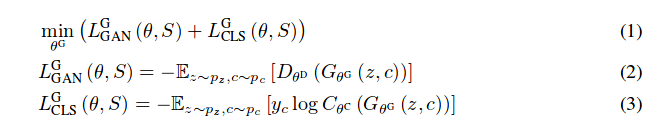where $$L^G_{GAN}(\theta, s)$$ and $$\lambda_{CLS}L^G_{CLS}(\theta, s)$$ are GAN loss and cross-entropy loss. $$y_c$$ is the one-hot encoding for the category c.

The category c is sampled uniformly and z is sampled from a Gaussian distribution.

The discriminator is trained according to the following equations: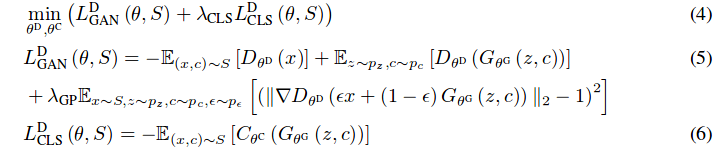Sequential fine tuning

The authors define a sequence of T tasks, one for each category. They then train their GAN one task at a time. Each GAN is initialized with the previous GAN’s parameters.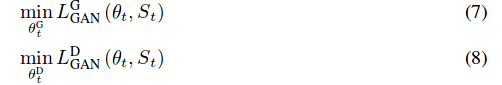Elastic Weight Consolidation

The authors present Elastic Weight Consolidation as a baseline for their new method to prevent forgetting. Elastic Weight Consolidation involves adding regularization to prevent the GAN’s parameters from changing too much during each task.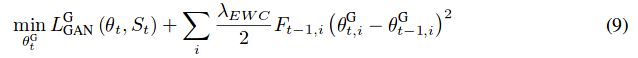$$F_{t-1,i}$$ is the Fisher information matrix that indicates how sensitive the parameter $$\theta^G_{t, i}$$ is to forgeting.

## Method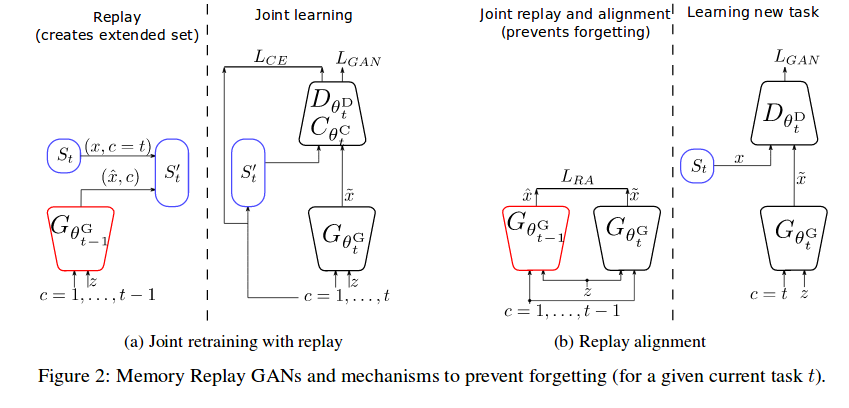Joint retraining with replayed samples

Their first method involves extending the current task’s dataset with the memory from the previous task’s GAN. $$S_t' = S_c \cup_{c\in{1,...,t-1}} \tilde{S_c}$$, where $$\tilde{S_c}$$ is the replay set generated by $$\tilde{x} = G_{\theta^G_{t-1}}(z, c)$$.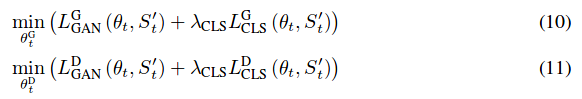Replay alignment

The second method the authors propose involves adding a loss term for the generator. This loss term is an L2 loss between the current and previous GAN outputs for the same category c and latent vector z.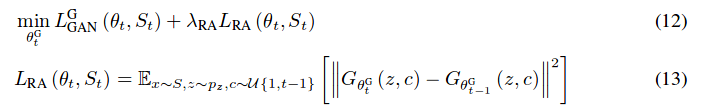## Results

The authors tested their two MeRGANs on digits generation with MNIST and SVHN and scene generation with 4 classes from the LSUN dataset. They compared their results to joint training, sequential fine tuning, Elastic Weight Consolidation and deep generative replay (Unconditional GAN with replay memory).

# MNIST and SVHN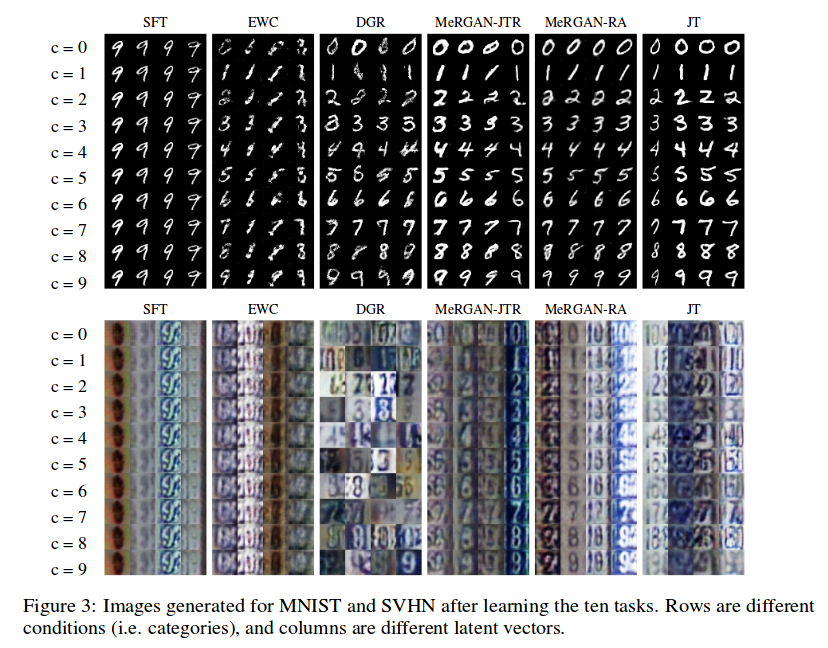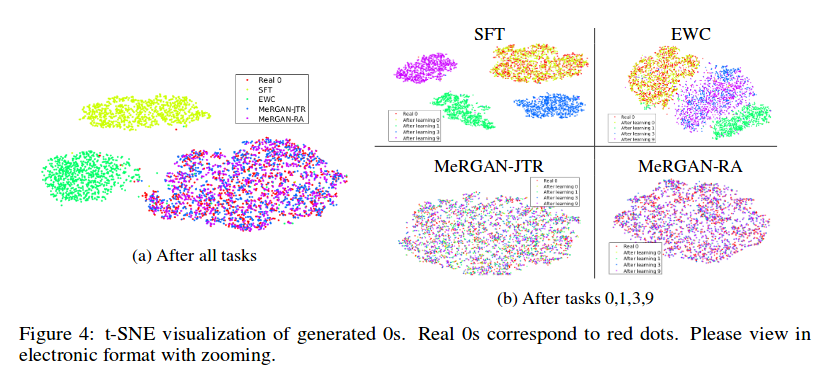The authors trained a classifier on real data and used the classification accuracy on the generated data to evaluate forgetting.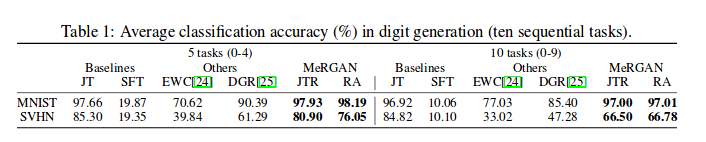# LSUN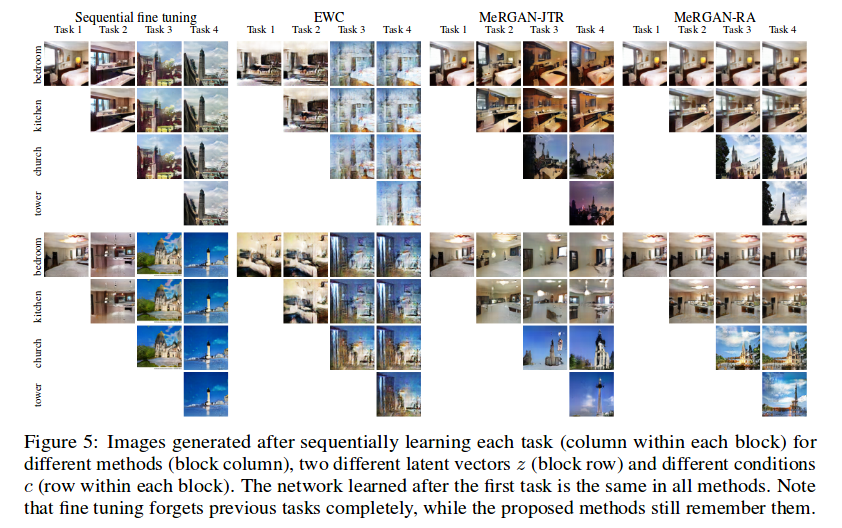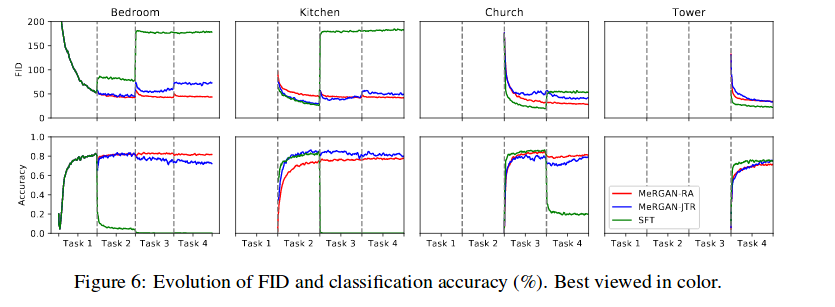The authors used a classifier to evaluate forgetting. They tested a classifier trained on real data on generated data and a classifier trained on generated data on real data (Rev acc.). Finally, they tested the Frechet Inception Distance (FID) on the generated data.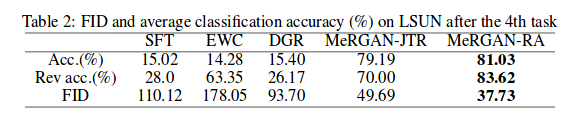Code: https://github.com/WuChenshen/MeRGAN